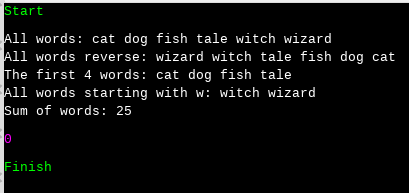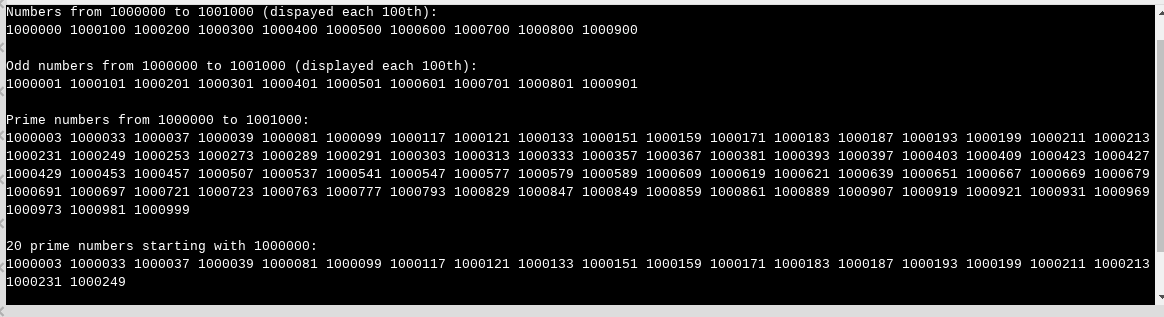# C++20: Functional Patterns with the Ranges Library

My last post C++20: The Ranges Library, gave you the first impression of the ranges library. Today’s post is about functional patterns: function composition and lazy evaluation. They become first-class citizens in C++20.The Standard Template Library (STL) algorithms are sometimes a little inconvenient. They need a beginning and an end iterator. This is often more than you want to write.

```#include <numeric>
#include <iostream>
#include <vector>

int main() {

std::vector<int> myVec{1, 2, 3, 4, 5, 6, 7, 8, 9};
auto res = std::accumulate(std::begin(myVec), std::end(myVec), 0);
// std::accumulate(myVec, 0);                                      // (1)
std::cout << res << std::endl;      //  45

}
```

Would it be nice if std::accumulate could be executed directly on the container, such as in line (2)?

##Modernes C++ Mentoring

Be part of my mentoring programs:

• "Fundamentals for C++ Professionals" (open)
• "Design Patterns and Architectural Patterns with C++" (open)
• "C++20: Get the Details" (reopens December 2023)
• Do you want to stay informed about my mentoring programs: Subscribe via E-Mail.

## Direct on the Container

The following program creates direct views on the keys (line 1) and the values (line  3) of the std::unordered_map

```// rangesEntireContainer.cpp

#include <iostream>
#include <ranges>
#include <string>
#include <unordered_map>

int main() {

std::unordered_map<std::string, int> freqWord{ {"witch", 25}, {"wizard", 33}, {"tale", 45},
{"dog", 4}, {"cat", 34}, {"fish", 23} };

std::cout << "Keys" << std::endl;
auto names = std::views::keys(freqWord);                                            // (1)
for (const auto& name : names){ std::cout << name << " "; };
std::cout << std::endl;
for (const auto& name : std::views::keys(freqWord)){ std::cout << name << " "; };   // (2)

std::cout << "\n\n";

std::cout << "Values: " << std::endl;
auto values = std::views::values(freqWord);                                          // (3)
for (const auto& value : values){ std::cout << value << " "; };
std::cout << std::endl;
for (const auto& value : std::views::values(freqWord)){ std::cout << value << " "; } // (4)

}
```

Of course, the keys and values can be displayed directly (lines 2 and line 4). The output is identical.Working directly on the container is not revolutionary, but function composition and lazy evaluation are.

## Function Composition

In my following example, I use std::map because the ordering of the keys is crucial.

```// rangesComposition.cpp

#include <iostream>
#include <ranges>
#include <string>
#include <map>

int main() {

std::map<std::string, int> freqWord{ {"witch", 25}, {"wizard", 33}, {"tale", 45},
{"dog", 4}, {"cat", 34}, {"fish", 23} };

std::cout << "All words: ";                     // (1)
for (const auto& name : std::views::keys(freqWord)) { std::cout << name << " "; };

std::cout << std::endl;

std::cout << "All words reverse: ";             // (2)
for (const auto& name : std::views::keys(freqWord) | std::views::reverse) { std::cout << name << " "; };

std::cout << std::endl;

std::cout << "The first 4 words: ";             // (3)
for (const auto& name : std::views::keys(freqWord) | std::views::take(4)) { std::cout << name << " "; };

std::cout << std::endl;

std::cout << "All words starting with w: ";     // (4)
auto firstw = [](const std::string& name){ return name == 'w'; };
for (const auto& name : std::views::keys(freqWord) | std::views::filter(firstw)) { std::cout << name << " "; };

std::cout << std::endl;
// (5)
auto lengthOf = [](const std::string& name){ return name.size(); };
auto res = ranges::accumulate(std::views::keys(freqWord) | std::views::transform(lengthOf), 0);
std::cout << "Sum of words: " << res << std::endl;

}
```

In this case, I’m only interested in the keys. I display all of them (line 1), all of them reversed (line 2), the first four (line 3), and the keys starting with the letter ‘w’ (line 4). Line (5) sums up all the lengths of all words.

The pipe symbol | is syntactic sugar for function composition. Instead of C(R), you can write R | C. Consequentially, the following two lines are equivalent.

```auto rev2 = std::views::reverse(ranges::views::keys(freqWord));auto rev = std::views::keys(freqWord) | ranges::views::reverse;
```

Finally, here is the output of the program.## Lazy Evaluation

I use in my example std::views::iota. This function is a range factory for creating a sequence of elements by successively incrementing an initial value. This sequence can be finite or infinite. The following example fills a std::vector with ten int’s, starting with 0.

```// rangesIota.cpp

#include <iostream>
#include <numeric>
#include <ranges>
#include <vector>

int main() {

std::cout << std::boolalpha;

std::vector<int> vec;
std::vector<int> vec2;

for (int i: std::views::iota(0, 10)) vec.push_back(i);                      // (1)

for (int i: std::views::iota(0) | std::views::take(10)) vec2.push_back(i);  // (2)

std::cout << "vec == vec2: " << (vec == vec2) << '\n'; // true

for (int i: vec) std::cout << i << " ";                // 0 1 2 3 4 5 6 7 8 9

}
```

The first iota call (line 1) creates all numbers from 0 to 9, incremented by 1. The second iota call (line 2) creates an infinite data stream, starting with 0 and incremented by 1. std::views::iota(0) is lazy. I only get a new value if I ask for it. I asked for it ten times. Consequentially, both vectors are identical.

Now,  I want to solve a small challenge. I want to find the first 20 prime numbers, starting with 1000000.

```// rangesLazy.cpp

#include <iostream>
#include <ranges>

bool isPrime(int i) {
for (int j=2; j*j <= i; ++j){
if (i % j == 0) return false;
}
return true;
}

int main() {
// (1)
std::cout << "Numbers from 1000000 to 1001000 (dispayed each 100th): " << std::endl;
for (int i: std::views::iota(1000000, 1001000)) {
if (i % 100 == 0) std::cout << i << " ";
}

std::cout << "\n\n";
// (2)
auto odd = [](int i){ return i % 2 == 1; };
std::cout << "Odd numbers from 1000000 to 1001000 (displayed each 100th): " << std::endl;
for (int i: std::views::iota(1000000, 1001000) | std::views::filter(odd)) {
if (i % 100 == 1) std::cout << i << " ";
}

std::cout << "\n\n";
// (3)
std::cout << "Prime numbers from 1000000 to 1001000: " << std::endl;
for (int i: std::views::iota(1000000, 1001000) | std::views::filter(odd)
| std::views::filter(isPrime)) {
std::cout << i << " ";
}

std::cout << "\n\n";
// (4)
std::cout << "20 prime numbers starting with 1000000: " << std::endl;
for (int i: std::views::iota(1000000) | std::views::filter(odd)
| std::views::filter(isPrime)
| std::views::take(20)) {
std::cout << i << " ";
}

}
```

Here is my iterative strategy:

1. Of course, I don’t know when I have 20 primes greater than 1000000. To be on the safe side, I create 1000 numbers. For obvious reasons, I displayed only each 100th.
2. I’m only interested in the odd numbers; therefore, I removed the even numbers.
3. Now, it’s time to apply the next filter. The predicate isPrime returns if a number is a prime. As you can see in the following screenshot, I was too eager. I got 75 primes.
4. Laziness is a virtue. I use std::iota as an infinite number factory, starting with 1000000, and ask precisely for 20 primes.## What’s next?

Python has many very nice functions, such as range, map, filter, reduce, and zip. And, of course, there is also the slice operator and list comprehension. These functions are eager in Python 2, but map, filter, and reduce are lazy in Python 3. I will try an experiment in my next post. Which functions can I implement with similar functionality in C++ by using the ranges library?

Thanks a lot to my Patreon Supporters: Matt Braun, Roman Postanciuc, Tobias Zindl, G Prvulovic, Reinhold Dröge, Abernitzke, Frank Grimm, Sakib, Broeserl, António Pina, Sergey Agafyin, Андрей Бурмистров, Jake, GS, Lawton Shoemake, Jozo Leko, John Breland, Venkat Nandam, Jose Francisco, Douglas Tinkham, Kuchlong Kuchlong, Robert Blanch, Truels Wissneth, Kris Kafka, Mario Luoni, Friedrich Huber, lennonli, Pramod Tikare Muralidhara, Peter Ware, Daniel Hufschläger, Alessandro Pezzato, Bob Perry, Satish Vangipuram, Andi Ireland, Richard Ohnemus, Michael Dunsky, Leo Goodstadt, John Wiederhirn, Yacob Cohen-Arazi, Florian Tischler, Robin Furness, Michael Young, Holger Detering, Bernd Mühlhaus, Matthieu Bolt, Stephen Kelley, Kyle Dean, Tusar Palauri, Dmitry Farberov, Juan Dent, George Liao, Daniel Ceperley, Jon T Hess, Stephen Totten, Wolfgang Fütterer, Matthias Grün, Phillip Diekmann, Ben Atakora, Ann Shatoff, Rob North, and Bhavith C Achar.

Thanks, in particular, to Jon Hess, Lakshman, Christian Wittenhorst, Sherhy Pyton, Dendi Suhubdy, Sudhakar Belagurusamy, Richard Sargeant, Rusty Fleming, John Nebel, Mipko, Alicja Kaminska, Slavko Radman, and David Poole.

 My special thanks to EmbarcaderoMy special thanks to PVS-StudioMy special thanks to Tipi.buildMy special thanks to Take Up Code## Seminars

I’m happy to give online seminars or face-to-face seminars worldwide. Please call me if you have any questions.

### Standard Seminars (English/German)

Here is a compilation of my standard seminars. These seminars are only meant to give you a first orientation.

• C++ – The Core Language
• C++ – The Standard Library
• C++ – Compact
• C++11 and C++14
• Concurrency with Modern C++
• Design Pattern and Architectural Pattern with C++
• Embedded Programming with Modern C++
• Generic Programming (Templates) with C++

#### New

• Clean Code with Modern C++
• C++20

### Contact Me

Modernes C++ Mentoring,Tags:
0 replies